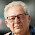## 15 September 2011

### How to Pronounce Hexadecimal Numbers

In 1999, the IEC introduced kibibyte and friends, because they were pissed off that kilobyte means 1000 bytes, but programmers wanted it to mean 1024 bytes and were insistently using it that way, correctness be damned. Unfortunately, nobody really gave a damn, and even though some things (Ubuntu for instance) show data sizes in KiB, nobody actually says “kibibyte”.

Someone asked recently on the Programmers Stack Exchange how hexadecimal numbers ought to be pronounced. In a valiant effort to pull an IEC and come up with a useful thing that nobody will use, here’s my system of pronunciation for hex numbers. The system is based on English (specifically American English) because it’s the lingua franca of programming. Enjoy.

Hexadecimal Number Name Decimal Equivalent
0x0000 0001 One 160 = 1
⋮
0x0000 000a A (ay) = ten 10
0x0000 000b B (bee) = eleven 11
0x0000 000c C (cee) = twelve 12
⋮
0x0000 0010 Tex 1 × 161 = 16
0x0000 0020 Twentex† = two tex 2 × 161 = 32
0x0000 0030 Thirtex† = three tex 3 × 161 = 48
0x0000 0040 Fortex† = four tex 4 × 161 = 64
0x0000 0050 Fiftex† = five tex 5 × 161 = 80
0x0000 0060 Sixtex = six tex 6 × 161 = 96
0x0000 0070 Seventex = seven tex 7 × 161 = 112
0x0000 0080 Eightex = eight tex 8 × 161 = 128
0x0000 0090 Ninetex = nine tex 9 × 161 = 144
0x0000 00a0 Tentex = ten tex 10 × 161 = 160
0x0000 00b0 Eleventex = eleven tex 11 × 161 = 176
⋮
0x0000 0100 One hundrex 162 = 256
0x0000 1000 One thousax 163 = 4096
0x0001 0000 Tex thousax = one thoutex‡ 164 = 65536
0x0010 0000 One hundrex thousax = tex thoutex 165 = 1048576
0x0100 0000 One milliox = one hundrex thoutex 166 = 16777216
0x1000 0000 One billiox†† = one thousax thoutex 169
⋮

† By analogy with “thirty”, “forty”, &c.

†† This system is based on the short scale—one billiox equals one thousax milliox, and one trilliox is one thousax billiox.

‡ I introduced the term “thoutex”, equal to 0x10000, because it’s more obvious that 0x0001 0000 is “one thoutex” rather than “tex thousax”: four-digit grouping makes more sense in hex, but three-digit grouping is the default in English, so I had to come up with something, and the hypothetical “thouten” sounded good in my mind to adapt.

Fractions are formed as in English, by adding -th: one texth, one hundrexth, one thousaxth, one thoutexth, &c.

Example: 0xa400f20.cd

• Ten hundrex fourtex thousax, fifteen hundrex twentex and twelvetex thirteen hundrexths
• Ay hundrex fourtex thousax, eff hundrex twentex and ceetex dee hundrexths
• Ay milliox, four hundrex thousax, eff hundrex twentex and ceetex dee hundrexths

Happy pronouncing! :)

#### 4 comments:

1.Eleventex? Surely you mean Aytex.

2.Anonymous: Aytex sounds too much like (i.e. identical to) "Eightex". In fact "Eight" sounds too close to "A" for comfort, but that aside if you chose something that didn't start with a T for the sixteens place, you could probably get away with it.

In fact, since it's Mexico's independence day on the day this was written, how about "mex"? :)

3.0x00a0 - Atex
0x00b0 - Betex
0x00c0 - Citex (Sitex)
0x00d0 - Detex
0x00e0 - Etex
0x00f0 - Fytex

for fractions, how about adding 'et' or maybe 'ette'? It much easier to pronounce and gives the impression of small.

4.In the 1960s I saw a paper in which someone presented a coherent system for pronouncing hexadecimal numbers. I probably have a printed copy of it around somewhere, but a cursory search for it online turned up nothing.